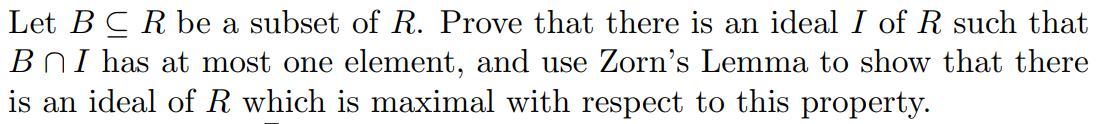Let BCR be a subset of R. Prove that there is an ideal I of R such thatB n I has at most one element, and use Zorn's Lemma to show that thereis an ideal of R which is maximal with respect to this property

Questionhelp_outlineImage TranscriptioncloseLet BCR be a subset of R. Prove that there is an ideal I of R such that B n I has at most one element, and use Zorn's Lemma to show that there is an ideal of R which is maximal with respect to this property fullscreen
Step 1

To show that ideals I and J  exist satisfying conditions 1) and 2)

Step 2

Recall the following basic definition.

Step 3

Thus, we have exhibited an ideal I whose inter...

Want to see the full answer?

See Solution

Want to see this answer and more?

Our solutions are written by experts, many with advanced degrees, and available 24/7

See Solution
Tagged in

Math